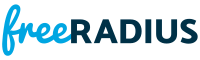heap.c File ReferenceInclude dependency graph for heap.c:

Go to the source code of this file.

Data Structures

struct  fr_heap_t

Macros

#define HEAP_LEFT(x)   ( 2*(x) + 1 )

#define HEAP_PARENT(x)   ( ( (x) - 1 ) / 2 )

#define HEAP_SWAP(a, b)   { void *_tmp = a; a = b; b = _tmp; }

#define RESET_OFFSET(heap, node)

#define SET_OFFSET(heap, node)

Functions

static int fr_heap_bubble (fr_heap_t *hp, size_t child)

fr_heap_tfr_heap_create (fr_heap_cmp_t cmp, size_t offset)

void fr_heap_delete (fr_heap_t *hp)

int fr_heap_extract (fr_heap_t *hp, void *data)

int fr_heap_insert (fr_heap_t *hp, void *data)

size_t fr_heap_num_elements (fr_heap_t *hp)

void * fr_heap_peek (fr_heap_t *hp)

Data Structure Documentation

 struct fr_heap_t

Definition at line 16 of file heap.c.

Data Fields
fr_heap_cmp_t cmp
size_t num_elements
size_t offset
void ** p
size_t size

Macro Definition Documentation

 #define HEAP_LEFT ( x ) ( 2*(x) + 1 )

Definition at line 30 of file heap.c.

 #define HEAP_PARENT ( x ) ( ( (x) - 1 ) / 2 )

Definition at line 29 of file heap.c.

 #define HEAP_SWAP ( a, b ) { void *_tmp = a; a = b; b = _tmp; }

Definition at line 32 of file heap.c.

 #define RESET_OFFSET ( heap, node )
Value:
if (heap->offset) \
*((int *)(((uint8_t *)heap->p[node]) + heap->offset)) = -1

Definition at line 88 of file heap.c.

 #define SET_OFFSET ( heap, node )
Value:
if (heap->offset) \
*((int *)(((uint8_t *)heap->p[node]) + heap->offset)) = node

Definition at line 80 of file heap.c.

Function Documentation

 static int fr_heap_bubble ( fr_heap_t * hp, size_t child )
static

Definition at line 118 of file heap.c.Here is the caller graph for this function:

 fr_heap_t* fr_heap_create ( fr_heap_cmp_t cmp, size_t offset )

Definition at line 44 of file heap.c.Here is the caller graph for this function:

 void fr_heap_delete ( fr_heap_t * hp )

Definition at line 36 of file heap.c.Here is the caller graph for this function:

 int fr_heap_extract ( fr_heap_t * hp, void * data )

Definition at line 147 of file heap.c.Here is the call graph for this function:Here is the caller graph for this function:

 int fr_heap_insert ( fr_heap_t * hp, void * data )

Definition at line 92 of file heap.c.Here is the call graph for this function:Here is the caller graph for this function:

 size_t fr_heap_num_elements ( fr_heap_t * hp )

Definition at line 217 of file heap.c.Here is the caller graph for this function:

 void* fr_heap_peek ( fr_heap_t * hp )

Definition at line 207 of file heap.c.Here is the caller graph for this function: